# The Diesel Engine

The diesel internal combustion engine differs from the gasoline powered Otto cycle by using a higher compression of the fuel to ignite the fuel rather than using a spark plug ("compression ignition" rather than "spark ignition").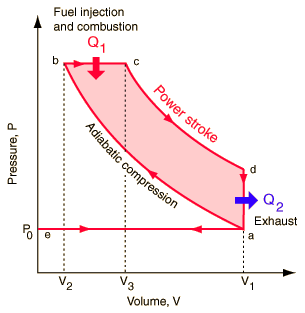Air standard diesel engine cycle In the diesel engine, air is compressed adiabatically with a compression ratio typically between 15 and 20. This compression raises the temperature to the ignition temperature of the fuel mixture which is formed by injecting fuel once the air is compressed. The ideal air-standard cycle is modeled as a reversible adiabatic compression followed by a constant pressure combustion process, then an adiabatic expansion as a power stroke and an isovolumetric exhaust. A new air charge is taken in at the end of the exhaust, as indicated by the processes a-e-a on the diagram.
 Since the compression and power strokes of this idealized cycle are adiabatic, the efficiency can be calculated from the constant pressure and constant volume processes. The input and output energies and the efficiency can be calculated from the temperatures and specific heats: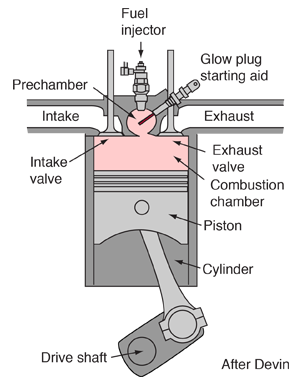It is convenient to express this efficiency in terms of the compression ratio rC = V1/V2 and the expansion ratio rE = V1/V3. The efficiency can be written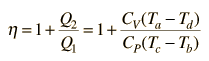and this can be rearranged to the form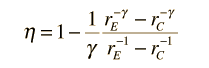Show

For an air standard engine with γ = 1.4 , compression ratio rC = 15 and expansion ratio rE = 5, this gives an ideal diesel efficiency of 56%.

For a diesel cycle for which γ =

with compression ratio rC =

and expansioin ratio rE = ,

the ideal efficiency for the cycle is η = %

Presuming that the intake pressure Pa is at atmospheric pressure, 101.3 kPa = 0 psig*, the adiabatic compression will produce a pressure

Pb = ParCγ = kPa = psig*.

The temperature produced by the compression is Tb = TarCγ-1

which for initial temperature Ta = K = °C

gives a temperature Tb = K = °C

The diesel cycle depends upon this temperature being high enough to ignite the fuel when it is injected.

*psig is pounds per square inch gauge pressure. Common pressure gauges in the U.S. measure pounds per square inch excess over atmospheric pressure.

 More details about the diesel cycle
Index

Heat engine concepts

Reference
Devins
Ch 4

 HyperPhysics***** Thermodynamics R Nave
Go Back

# Diesel Engine Theoretical EfficiencyAir standard diesel engine cycle Since the compression and power strokes of this idealized cycle are adiabatic, the efficiency can be calculated from the constant pressure and constant volume processes. The input and output energies and the efficiency can be calculated from the temperatures and specific heats:It is convenient to express this efficiency in terms of the compression ratio rC = V1/V2 and the expansion ratio rE = V1/V3.

The efficiency can be expressed in terms of the specific heats and temperatures.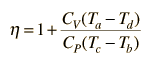Now using the ideal gas law PV=nRT and γ = CP/CV, this can be writtenNow using the fact that Va=Vd=V1 and Pc=Pb from the diagramDividing the numerator and denominator by V1Pc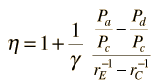Now making use of the adiabatic condition PVγ = constant,the efficiency can be writtenCalculation
Index

Heat engine concepts

References
Devins
Ch 4

Wark and Richards
Ch 15

 HyperPhysics***** Thermodynamics R Nave
Go Back

# Diesel Engine CycleAir standard diesel engine cycle

The term "compression ignition" is typically used in technical literature to describe the modern engines commonly called "Diesel engines". This is in contrast to "spark ignition" for the typical automobile gasoline engines that operate on a cycle derived from the Otto cycle. Rudolph Diesel patented the compression-ignition cycle which bears his name in the 1890s.Calculation
Index

Heat engine concepts

References
Devins
Ch 4

Wark and Richards
Ch 15

 HyperPhysics***** Thermodynamics R Nave
Go Back